# The ARIMA Procedure

### IDENTIFY Statement

• IDENTIFY VAR=variable options;

The IDENTIFY statement specifies the time series to be modeled, differences the series if desired, and computes statistics to help identify models to fit. Use an IDENTIFY statement for each time series that you want to model.

If other time series are to be used as inputs in a subsequent ESTIMATE statement, they must be listed in a CROSSCORR= list in the IDENTIFY statement.

The following options are used in the IDENTIFY statement. The VAR= option is required.

ALPHA=significance-level

The ALPHA= option specifies the significance level for tests in the IDENTIFY statement. The default is 0.05.

CENTER

centers each time series by subtracting its sample mean. The analysis is done on the centered data. Later, when forecasts are generated, the mean is added back. Note that centering is done after differencing. The CENTER option is normally used in conjunction with the NOCONSTANT option of the ESTIMATE statement.

CLEAR

deletes all old models. This option is useful when you want to delete old models so that the input variables are not prewhitened. (See the section Prewhitening for more information.)

CROSSCORR=variable (d11, d12, …, d1k )

CROSSCORR= (variable (d11, d12, …, d1k )... variable (d21, d22, …, d2k ))

names the variables cross-correlated with the response variable given by the VAR= specification.

Each variable name can be followed by a list of differencing lags in parentheses, the same as for the VAR= specification. If differencing is specified for a variable in the CROSSCORR= list, the differenced series is cross-correlated with the VAR= option series, and the differenced series is used when the ESTIMATE statement INPUT= option refers to the variable.

DATA=SAS-data-set

specifies the input SAS data set that contains the time series. If the DATA= option is omitted, the DATA= data set specified in the PROC ARIMA statement is used; if the DATA= option is omitted from the PROC ARIMA statement as well, the most recently created data set is used.

ESACF

computes the extended sample autocorrelation function and uses these estimates to tentatively identify the autoregressive and moving-average orders of mixed models.

The ESACF option generates two tables. The first table displays extended sample autocorrelation estimates, and the second table displays probability values that can be used to test the significance of these estimates. The P=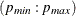and Q=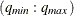options determine the size of the table.

The autoregressive and moving-average orders are tentatively identified by finding a triangular pattern in which all values are insignificant. The ARIMA procedure finds these patterns based on the IDENTIFY statement ALPHA= option and displays possible recommendations for the orders.

The following code generates an ESACF table with dimensions of p=(0:7) and q=(0:8).

   proc arima data=test;
identify var=x esacf p=(0:7) q=(0:8);
run;


See the section The ESACF Method for more information.

MINIC

uses information criteria or penalty functions to provide tentative ARMA order identification. The MINIC option generates a table that contains the computed information criterion associated with various ARMA model orders. The PERROR=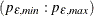option determines the range of the autoregressive model orders used to estimate the error series. The P=and Q=options determine the size of the table. The ARMA orders are tentatively identified by those orders that minimize the information criterion.

The following statements generate a MINIC table with default dimensions of p=(0:5) and q=(0:5) and with the error series estimated by an autoregressive model with an order,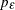, that minimizes the AIC in the range from 8 to 11.

   proc arima data=test;
identify var=x minic perror=(8:11);
run;


See the section The MINIC Method for more information.

NLAG=number

indicates the number of lags to consider in computing the autocorrelations and cross-correlations. To obtain preliminary estimates of an ARIMA(p, d, q ) model, the NLAG= value must be at least p +q +d. The number of observations must be greater than or equal to the NLAG= value. The default value for NLAG= is 24 or one-fourth the number of observations, whichever is less. Even though the NLAG= value is specified, the NLAG= value can be changed according to the data set.

NOMISS

uses only the first continuous sequence of data with no missing values. By default, all observations are used.

NOPRINT

suppresses the normal printout (including the correlation plots) generated by the IDENTIFY statement.

OUTCOV=SAS-data-set

writes the autocovariances, autocorrelations, inverse autocorrelations, partial autocorrelations, and cross covariances to an output SAS data set. If the OUTCOV= option is not specified, no covariance output data set is created. See the section OUTCOV= Data Set for more information.

P=(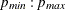)

see the ESACF, MINIC, and SCAN options for details.

PERROR=(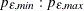)

determines the range of the autoregressive model orders used to estimate the error series in MINIC, a tentative ARMA order identification method. See the section The MINIC Method for more information. By default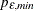is set to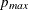and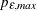is set to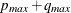, whereand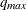are the maximum settings of the P= and Q= options on the IDENTIFY statement.

Q=(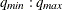)

see the ESACF, MINIC, and SCAN options for details.

SCAN

computes estimates of the squared canonical correlations and uses these estimates to tentatively identify the autoregressive and moving-average orders of mixed models.

The SCAN option generates two tables. The first table displays squared canonical correlation estimates, and the second table displays probability values that can be used to test the significance of these estimates. The P=and Q=options determine the size of each table.

The autoregressive and moving-average orders are tentatively identified by finding a rectangular pattern in which all values are insignificant. The ARIMA procedure finds these patterns based on the IDENTIFY statement ALPHA= option and displays possible recommendations for the orders.

The following code generates a SCAN table with default dimensions of p=(0:5) and q=(0:5). The recommended orders are based on a significance level of 0.1.

   proc arima data=test;
identify var=x scan alpha=0.1;
run;


See the section The SCAN Method for more information.

STATIONARITY=

performs stationarity tests. Stationarity tests can be used to determine whether differencing terms should be included in the model specification. In each stationarity test, the autoregressive orders can be specified by a range, test=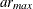, or as a list of values, test=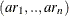, where test is ADF, PP, or RW. The default is (0,1,2).

See the section Stationarity Tests for more information.

STATIONARITY=(ADF= AR orders DLAG= s )
STATIONARITY=(DICKEY= AR orders DLAG= s )

performs augmented Dickey-Fuller tests. If the DLAG=option is specified withis greater than one, seasonal Dickey-Fuller tests are performed. The maximum allowable value ofis 12. The default value ofis 1. The following code performs augmented Dickey-Fuller tests with autoregressive orders 2 and 5.

   proc arima data=test;
identify var=x stationarity=(adf=(2,5));
run;

STATIONARITY=(PP= AR orders )
STATIONARITY=(PHILLIPS= AR orders )

performs Phillips-Perron tests. The following statements perform augmented Phillips-Perron tests with autoregressive orders ranging from 0 to 6.

   proc arima data=test;
identify var=x stationarity=(pp=6);
run;

STATIONARITY=(RW=AR orders )
STATIONARITY=(RANDOMWALK=AR orders )

performs random-walk-with-drift tests. The following statements perform random-walk-with-drift tests with autoregressive orders ranging from 0 to 2.

   proc arima data=test;
identify var=x stationarity=(rw);
run;

VAR=variable
VAR= variable ( d1, d2, …, dk )

names the variable that contains the time series to analyze. The VAR= option is required.

A list of differencing lags can be placed in parentheses after the variable name to request that the series be differenced at these lags. For example, VAR=X(1) takes the first differences of X. VAR=X(1,1) requests that X be differenced twice, both times with lag 1, producing a second difference series, which is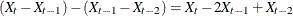.

VAR=X(2) differences X once at lag two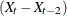.

If differencing is specified, it is the differenced series that is processed by any subsequent ESTIMATE statement.

WHITENOISE=ST | IGNOREMISS

specifies the type of test statistic that is used in the white noise test of the series when the series contains missing values. If WHITENOISE=IGNOREMISS, the standard Ljung-Box test statistic is used. If WHITENOISE=ST, a modification of this statistic suggested by Stoffer and Toloi (1992) is used. The default is WHITENOISE=ST.